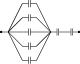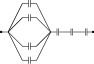# Capacitors in Series and Parallel

## Problems from IIT JEE

Problem (IIT JEE 1990): Seven capacitors each of capacitance ${2}\;\mathrm{\mu F}$ are connected in a configuration to obtain an effective capacitance ${\frac{10}{11}}\;\mathrm{\mu F}$. Which of the following combination will achieve the desired result?

1.2.3.4.Solution: In option A, equivalent capacitance of five capacitors in parallel is $C_1={10}\;\mathrm{\mu F}$ and that of two capacitors in series is $C_2={1}\;\mathrm{\mu F}$. The $C_1$ and $C_2$ in series give equivalent capacitance $C_\text{eq}=\frac{C_1C_2}{C_1+C_2}={\frac{10}{11}}\;\mathrm{\mu F}$.